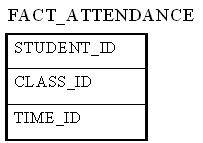# Factless Fact Table

Data Warehousing > Concepts > Factless Fact Table

A factless fact table is a fact table that does not have any measures. It is essentially an intersection of dimensions. On the surface, a factless fact table does not make sense, since a fact table is, after all, about facts. However, there are situations where having this kind of relationship makes sense in data warehousing.

For example, think about a record of student attendance in classes. In this case, the fact table would consist of 3 dimensions: the student dimension, the time dimension, and the class dimension. This factless fact table would look like the following:The only measure that you can possibly attach to each combination is "1" to show the presence of that particular combination. However, adding a fact that always shows 1 is redundant because we can simply use the COUNT function in SQL to answer the same questions.

Factless fact tables offer the most flexibility in data warehouse design. For example, one can easily answer the following questions with this factless fact table:

• How many students attended a particular class on a particular day?
• How many classes on average does a student attend on a given day?

Without using a factless fact table, we will need two separate fact tables to answer the above two questions. With the above factless fact table, it becomes the only fact table that's needed.

Next Page: Junk Dimension